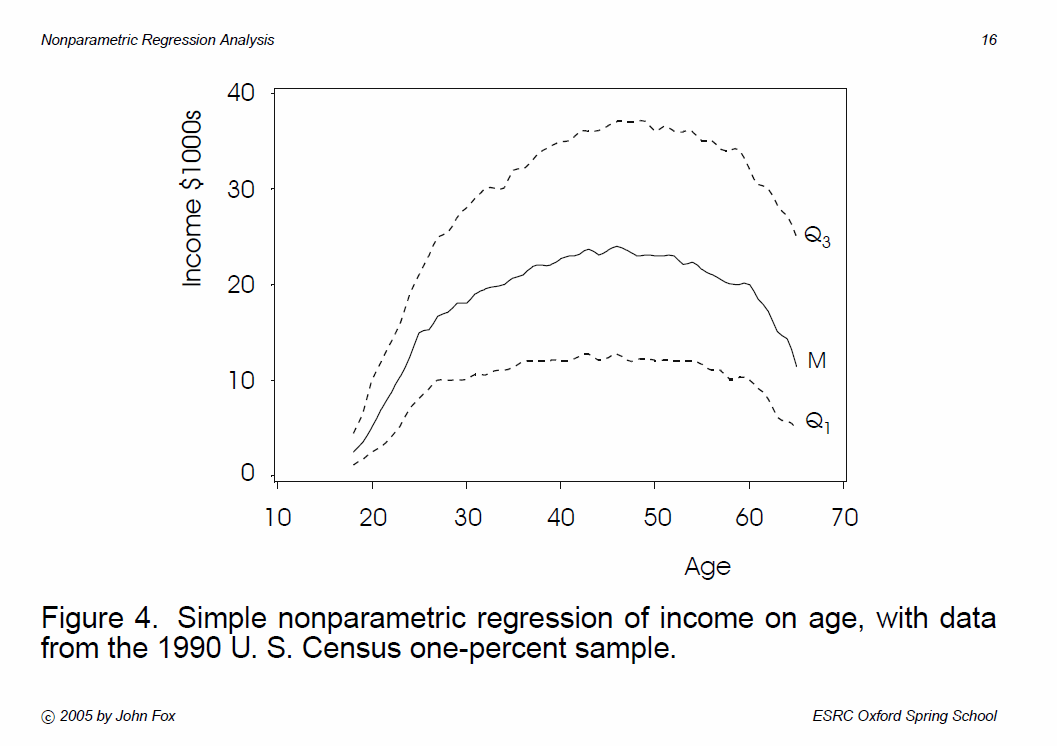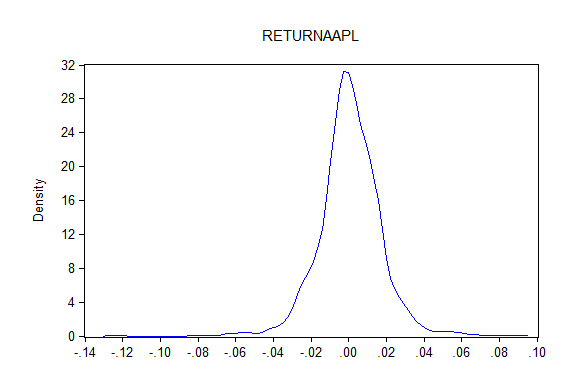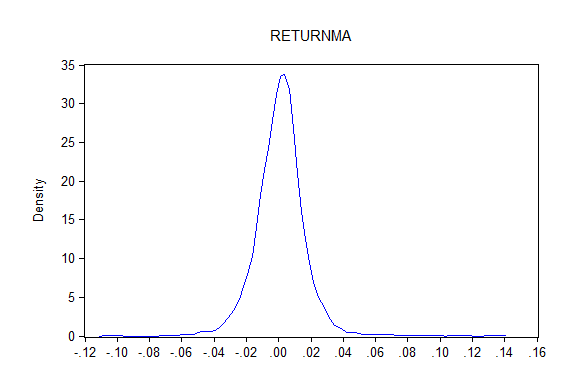1
Dec 16

## Nonparametric estimation for AP Stats

### Nonparametric estimation is the right topic for expanding the Stats agendaFigure 1. Dependence of income on age

For the last several years I have been doing research in nonparametric estimation. It is intellectually rewarding and it is the best tool to show Stats students the usefulness of Statistics. Agresti and Franklin have a chapter on nonparametric estimation. However, the choice of the topics (Wilcoxon test and Kruskal-Wallis test) is unfortunate. These two tests are about comparing means from two samples. They provide just numbers (corresponding statistics), which is not very appealing because the students see just another solution to the familiar problem.

Nonparametric technique is best in nonlinear curve fitting, and this is its selling point because it is VISUAL. The following examples explain the difference between parametric and nonparametric estimation.

### Example 1

Suppose we want to use simple regression to estimate dependence of consumption on income. This is a parametric model, with two parameters (intercept and slope). Suppose the fitted line is$Consumption=0.1+0.9\times Income$ (I just put plausible numbers). The slope$0.9$ is interpreted as the marginal propensity to consume and can be used in economic modeling to find the budget multiplier. The advantage of parametric estimation is that often estimated parameters have economic meaning.

### Example 2

This example has been taken from Lecture Notes by John Fox. Now let us look at dependence of income on age. It is clear that income is low for young people, then rises with age until middle age and declines after retirement. The dependence is obviously nonlinear and, a priori, no guesses can be made about the shape of the curve.

Figure 1 shows the median and quartiles of the distribution of income from wages and salaries as a function of single years of age. The data are taken from the 1990 U.S. Census one-percent Public Use Microdata Sample, and represent 1.24 million observations. Income starts increasing at around 18 years, tops out at 48 and declines till the age of 65. The fitted line is approximately linear until the age of 24, so young people enjoy a highest and constant income growth rate.

### Example 3Figure 2. Density of return on Apple stockFigure 3. Density of return on MA stock

What would have been the better 5-year investment: Apple or MasterCard? Figure 2 shows that the density of return on Apple stock has a negative mode. The density of return on MasterCard has the mode close to zero. This tells us that MasterCard would be better. Indeed, the annual return on Apple is 18%, while on MasterCard it is 29% (over the last 5 years). Nonparametric estimates of densities (kernel density estimates) are used by financial analysts to simulate stock prices to predict their future movements.

Remark. For simple statistical tasks I recommend Eviews student version for two reasons. 1) It has excellent Help. When I want my students to understand just the essence and avoid proofs, I tell them to read Eviews Help. 2) The student version is just \$39.95. Figures 2 and 3 have been produced using Eviews.